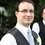# Nature of roots of a quartic

I wonder if anyone can answer my question posted to the top-rated solution?

In case the link doesn't work, the given solution said:

"Let p(x)=x4−2x3−9x2+2x+8 and q(x)=ax+b. By sketching the graph of p, we can easily see that the only way that q can be tangent to p in two distinct points is if q is right at the bottom of p, touching each 2 down-humps in a different point, let us say, c and d.

Now let us sketch r(x)=p(x)−q(x). Because q is right at the bottom of p, the difference p(x)−q(x) is always positive, except for x=c and x=d, which make it go to zero. Therefore, r is a polynomial with only 2 real roots c and d. Furthermore, r is always positive when we get closer to c and to d, which means both have multiplicity 2 or greater."

and I am looking for explanation of the last sentence.Note by Matt McNabb
6 years, 8 months ago

This discussion board is a place to discuss our Daily Challenges and the math and science related to those challenges. Explanations are more than just a solution — they should explain the steps and thinking strategies that you used to obtain the solution. Comments should further the discussion of math and science.

When posting on Brilliant:

• Use the emojis to react to an explanation, whether you're congratulating a job well done , or just really confused .
• Ask specific questions about the challenge or the steps in somebody's explanation. Well-posed questions can add a lot to the discussion, but posting "I don't understand!" doesn't help anyone.
• Try to contribute something new to the discussion, whether it is an extension, generalization or other idea related to the challenge.

MarkdownAppears as
*italics* or _italics_ italics
**bold** or __bold__ bold
- bulleted- list
• bulleted
• list
1. numbered2. list
1. numbered
2. list
Note: you must add a full line of space before and after lists for them to show up correctly
paragraph 1paragraph 2

paragraph 1

paragraph 2

[example link](https://brilliant.org)example link
> This is a quote
This is a quote
    # I indented these lines
# 4 spaces, and now they show
# up as a code block.

print "hello world"
# I indented these lines
# 4 spaces, and now they show
# up as a code block.

print "hello world"
MathAppears as
Remember to wrap math in $$ ... $$ or $ ... $ to ensure proper formatting.
2 \times 3 $2 \times 3$
2^{34} $2^{34}$
a_{i-1} $a_{i-1}$
\frac{2}{3} $\frac{2}{3}$
\sqrt{2} $\sqrt{2}$
\sum_{i=1}^3 $\sum_{i=1}^3$
\sin \theta $\sin \theta$
\boxed{123} $\boxed{123}$

Sort by:

Note that the url that you get for the problem is customized to you. In order for others to view it, you have to use the url provided in "Share this problem". I have updated the link that you provided in this discussion.

Staff - 6 years, 8 months ago

Thanks Calvin. I wasn't sure if it was or not.

- 6 years, 8 months ago